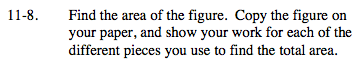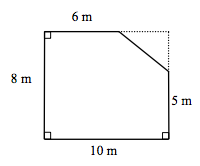### Home > MC2 > Chapter Ch11 > Lesson 11.1.1 > Problem11-8

11-8.

Find the area of the figure. Copy the figure on your paper, and show your work for each of the different pieces you use to find the total area. Homework Help ✎The shape to the right is missing a triangular segment in the top right corner.

With the inclusion of the triangular piece, the shape would form a rectangle.

Now solve for the area of the figure above by finding the area of the complete rectangle minus the area of the missing triangle piece.

With this information, we can solve for the lengths of the missing triangular piece:

10 − 6 = 4
8 − 5 = 3

80 m2 − 6m2 = 74 m2